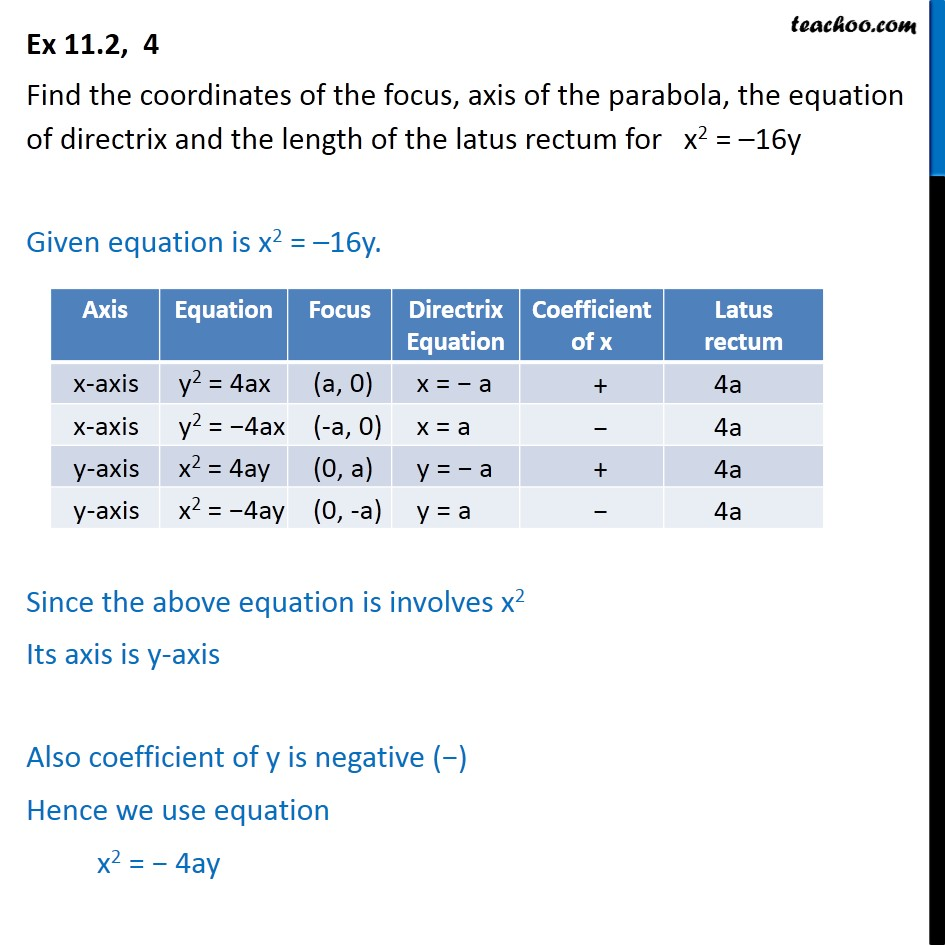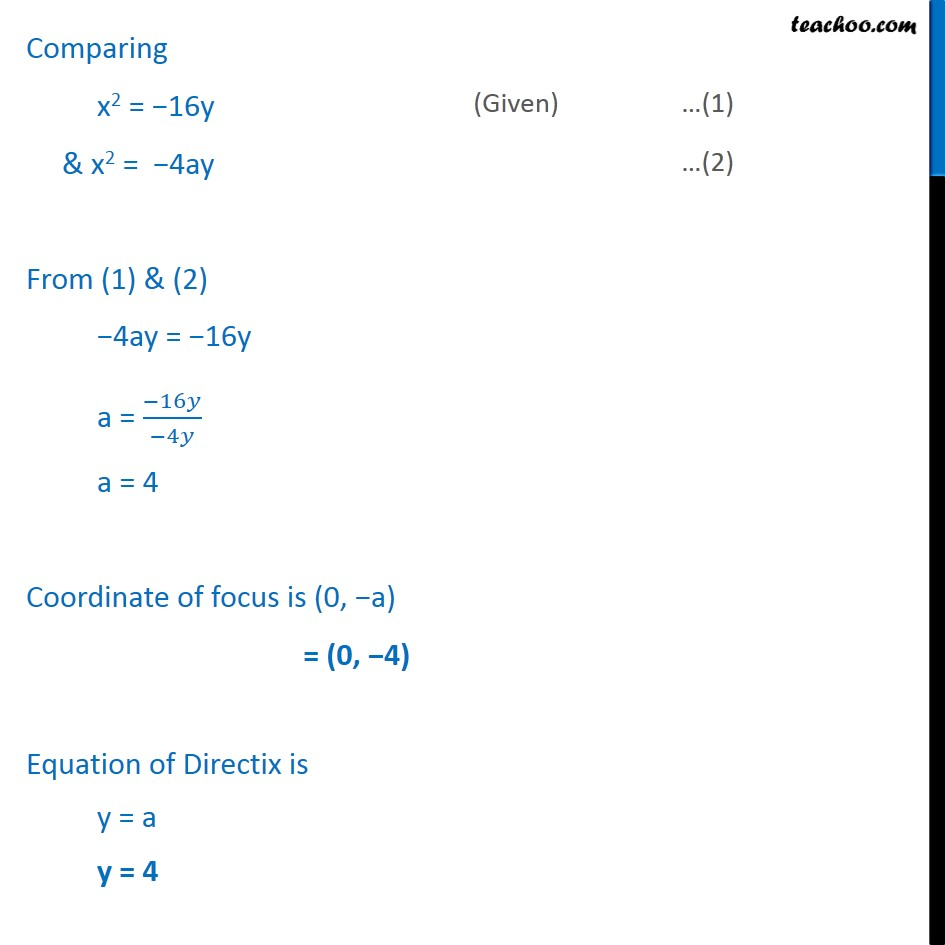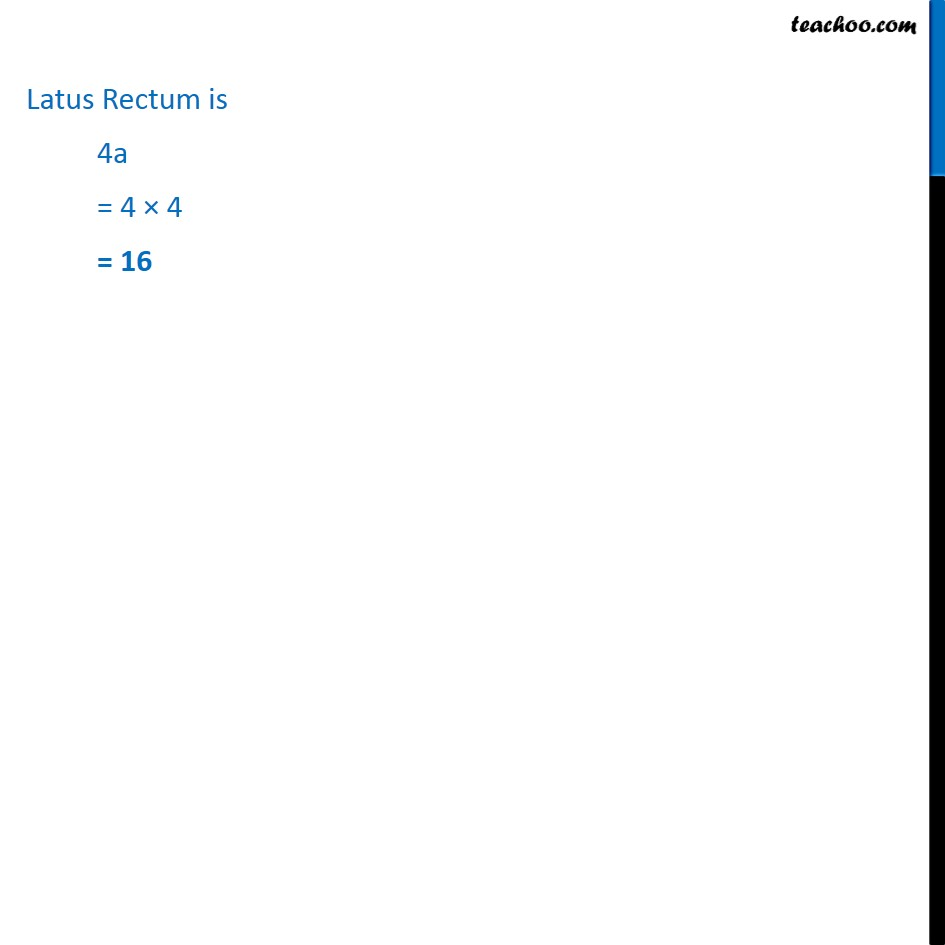1. Chapter 11 Class 11 Conic Sections
2. Serial order wise
3. Ex 11.2

Transcript

Ex 11.2, 4 Find the coordinates of the focus, axis of the parabola, the equation of directrix and the length of the latus rectum for x2 = 16y Given equation is x2 = 16y. Since the above equation is involves x2 Its axis is y-axis Also coefficient of y is negative ( ) Hence we use equation x2 = 4ay Latus Rectum is 4a = 4 4 = 16

Ex 11.2

About the AuthorDavneet Singh
Davneet Singh is a graduate from Indian Institute of Technology, Kanpur. He has been teaching from the past 9 years. He provides courses for Maths and Science at Teachoo.